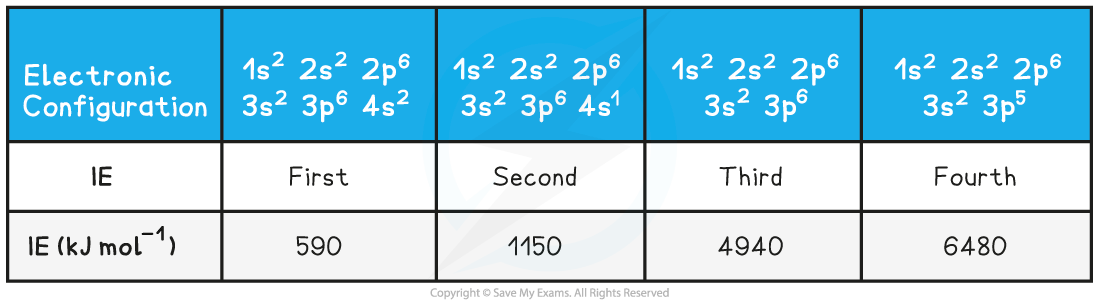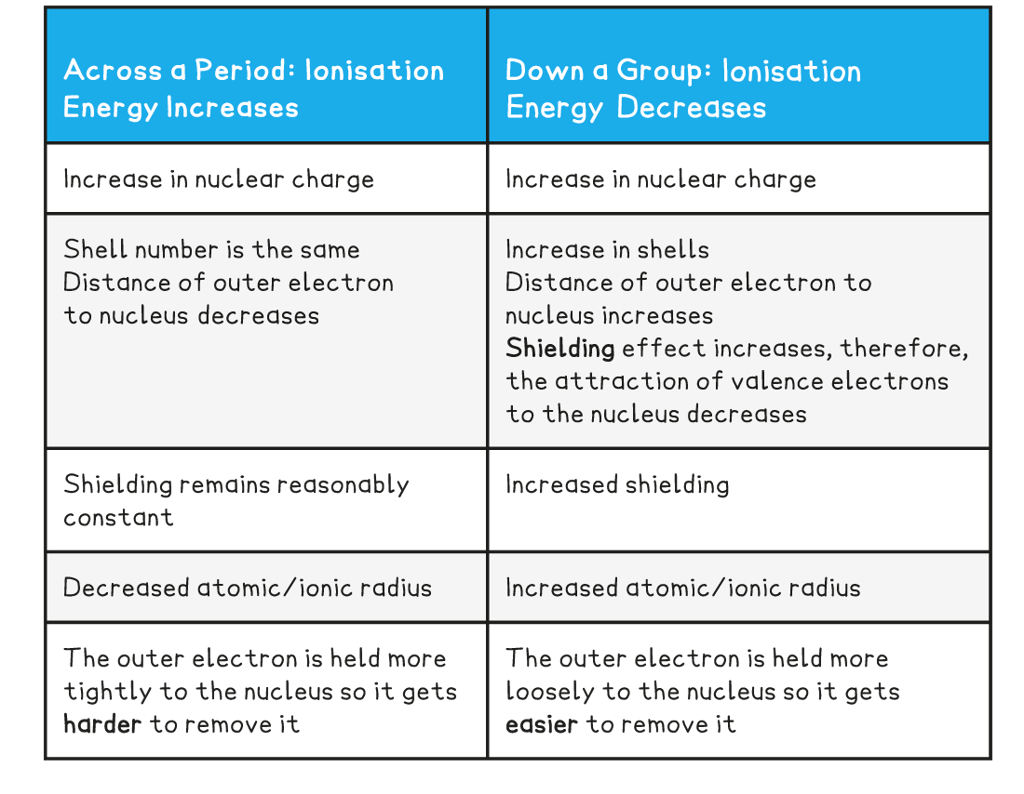# Edexcel A Level Chemistry:复习笔记1.2.1 Ionisation Energy

### Factors Affecting Ionisation Energy

#### Ionisation energy

• The ionisation Energy (IE) of an element is the amount of energy required to remove one mole of electrons from one mole of gaseous atoms of an element to form one mole of gaseous ions
• Ionisation energies are measured under standard conditions which are 298 K and 101 kPa
• The units of IE are kilojoules per mole (kJ mol-1)
• The first ionisation energy (IE1) is the energy required to remove one mole of electrons from one mole of gaseous atoms of an element to form one mole of gaseous 1+ ions
• E.g. the first ionisation energy of gaseous calcium:

Ca (g) → Ca+ (g) + e-          IE1 = +590 kJ mol-1

#### Successive ionisation energies of an element

• More than one electron can be removed form an atom and each time you remove an electron there is a successive ionisation energy
• These are called second, third ionisation energy and so on
• The second ionisation energy (IE2) is defined as
• is the energy required to remove one mole of electrons from one mole of gaseous 1+ ions to form one mole of gaseous 2+ ions
• And can be represented as can be represented as

X+ (g) → X2+ (g) + e-

OR (for calcium)

Ca+ (g) → Ca2+ (g) + e-  IE2 = 1145 kJ mol-1

• The third ionisation energy (IE3) of an element can be represented as

X2+ (g) → X3+ (g) + e-

OR (for calcium)

Ca2+ (g) → Ca3+ (g) + e-  IE3 = 4912 kJ mol-1

• The successive ionisation energies of an element increase
• This is because once you have removed the outer electron from an atom, you have formed a positive ion
• Removing an electron from a positive ion is more difficult than from a neutral atom
• As more electrons are removed, the attractive forces increase due to decreasing shielding and an increase in the proton to electron ratio
• The increase in ionisation energy, however, is not constant and is dependent on the atom’s electronic configuration
• Taking calcium as an example:

Ionisation Energies of Calcium Table### First Ionisation Energy - Period

#### Trends in Ionisation Energies

• Ionisation energies show periodicity - a trend across a period of the Periodic Table
• As could be expected from their electron configuration, the group 1 metals have a relatively low ionisation energy, whereas the noble gases have very high ionisation energies
• The size of the first ionisation energy is affected by four factors:
• Size of the nuclear charge
• Distance of outer electrons from the nucleus
• Shielding effect of inner electrons
• Spin-pair repulsion

#### Ionisation energy across a period

• The ionisation energy across a period generally increases due to the following factors:
• Across a period the nuclear charge increases
• This causes the atomic radius of the atoms to decrease, as the outer shell is pulled closer to the nucleus, so the distance between the nucleus and the outer electrons decreases
• The shielding by inner shell electrons remain reasonably constant as electrons are being added to the same shell
• It becomes harder to remove an electron as you move across a period; more energy is needed
• So, the ionisation energy increases

#### Dips in the trend

• There is a slight decrease in IE1 between beryllium and boron as the fifth electron in boron is in the 2p subshell, which is further away from the nucleus than the 2s subshell of beryllium
• Beryllium has a first ionisation energy of 900 kJ mol-1 as its electron configuration is 1s2 2s2
• Boron has a first ionisation energy of 800 kJ mol-1 as its electron configuration is 1s2 2s2 2px1

• There is a slight decrease in IE1 between nitrogen and oxygen due to spin-pair repulsion in the 2px orbital of oxygen
• Nitrogen has a first ionisation energy of 1400 kJ mol-1 as its electron configuration is 1s2 2s2 2px1 2py1 2pz1
• Oxygen has a first ionisation energy of 1310 kJ mol-1 as its electron configuration is 1s2 2s2 2px2 2py1 2pz1
• In oxygen, there are 2 electrons in the 2px orbital, so the repulsion between those electrons makes it slightly easier for one of those electrons to be removed

#### From one period to the next

• There is a large decrease in ionisation energy between the last element in one period, and the first element in the next period
• This is because:
• There is increased distance between the nucleus and the outer electrons as you have added a new shell
• There is increased shielding by inner electrons because of the added shell
• These two factors outweigh the increased nuclear charge

### First Ionisation Energy - Group

#### Ionisation energy down a group

• The ionisation energy down a group decreases due to the following factors:
• The number of protons in the atom is increased, so the nuclear charge increases
• But, the atomic radius of the atoms increases as you are adding more shells of electrons, making the atoms bigger
• So, the distance between the nucleus and outer electron increases as you descend the group
• The shielding by inner shell electrons increases as there are more shells of electrons
• These factors outweigh the increased nuclear charge, meaning it becomes easier to remove the outer electron as you descend a group
• So, the ionisation energy decreases

Ionisation Energy Trends across a Period & going down a Group Table